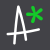# First a quick tour of the jupyter notebook¶

• Shortcuts
• Getting help
• Cell types
• Executing cells

### Formatted text¶

We can write formated text in Markdown cells (Esc + m) and there are plenty of resources available:

Unordered list

• item 1
• item 2
• sub-item a
• sub-item b
• item 3

Ordered list

1. first
2. second
3. third

italic, bold, underline (not strictly markdown but works in the notebook)

quoted text

SyntaxDescription
ParagraphText

### Equations¶

Mathematical expressions in notebooks use LaTeX: overleaf.com/learn/latex/Mathematical_expressions

Inline Gardner's equation $\rho = \alpha {V_P}^{\beta}$

Centered equation Darcy'y Law

$q = -\frac{k}{\mu L} \Delta p$

### Code¶

in-line code

and multiline formatted code:

# Some formatted python code

def say_hi():
"""Just print hello!"""
print('Hello!')
return None

### Code output¶

print('Hello Transfrom 2022!')
Hello Transfrom 2022!


### Images¶# A gentle introduction to python¶

### mathematical operators¶

# Live coding of basic mathematical operators


# Press shift+enter to execute a cell
2 + 3
5
2 + 3.5
5.5
15.5 - 76
-60.5
45 * 0.4
18.0
23 / 2
11.5
# Long division
23 // 2
11
# Remainder of long division: the "modulo operator"
23 % 2
1
2**3
8

### logical operators¶

# Live coding of logical operators


23 > 4
True
534 < 100
False
100 <= 100
True
200 >= 199
True
13 == 15
False
43 != 49
True
# Combining them
11 % 2 == 0
False
(4 > 2) and (50 <= 10)
False
(4 > 2) or (50 <= 10)
True

### Assignment¶

x = 10
# no output!
x
10

## Stepping sideways out of your comfort zone¶

Words and collections in python (i.e. str, list, dict)

### str¶

word = 'python'
type(word)
str
word
'python'
# single vs double quotes


double_quotes = "same thing"
double_quotes
'same thing'
len(word)
6
# str methods


word.upper()
'PYTHON'
word
'python'

### list¶

word_list = ['python', 'geology', 'programming', 'code', 'outcrop']
# again, no output, assignment is silent
# exploring lists


type(word_list)
list
len(word_list)
5
word_list
['python', 'geology', 'programming', 'code', 'outcrop']
'geology' in word_list
True
word_list.append('mineral')
# no output
word_list
['python', 'geology', 'programming', 'code', 'outcrop', 'mineral']

## Indexing and slicing¶

We can reach into these collections, there are two main things to remember:

1. python starts counting at 0
2. we use square brackets []

### Indexing and slicing str¶

len(word)
6
word
'python'
word
'p'
word
'n'
# half-open interval
word[0:3]
'pyt'

### Indexing and slicing list¶

# same thing with list
word_list
['python', 'geology', 'programming', 'code', 'outcrop', 'mineral']
word_list
'python'
word_list[-1]
'mineral'
word_list[4:]
['outcrop', 'mineral']

### dict¶

container = {'words': word_list, 'word': word, 'transform': 2022}
container
{'words': ['python', 'geology', 'programming', 'code', 'outcrop', 'mineral'], 'word': 'python', 'transform': 2022}
# Exploring python dict


container.keys()
dict_keys(['words', 'word', 'transform'])
container.values()
dict_values([['python', 'geology', 'programming', 'code', 'outcrop', 'mineral'], 'python', 2022])
container.get('word')
'python'

#### Importing more functions¶

Sometimes we need to reach for some part of python that isn't loaded by default, there are three major ways to import packages:

• import package -> imports everything. Access things using package.function().
• import package as pkg -> imports everything with an alias. Accessing things using pkg.function().
• from package import function1, function2 -> import only function1 and function2. Accessed as function1() and function2().

Here we use the third method to import the datetime object from the datetime library:

from datetime import datetime

And we can now update the dict with a new value, for example today's date using the datetime object we just imported:

# using datetime to add today's date to the dict


# Build this up
container.update({'Location': 'Global', 'date': datetime.now().date().isoformat()})
container
{'words': ['python', 'geology', 'programming', 'code', 'outcrop', 'mineral'], 'word': 'python', 'transform': 2022, 'Location': 'Global', 'date': '2022-04-07'}
container['date']
'2022-04-07'

### Keying into a dict¶

container.keys()
dict_keys(['words', 'word', 'transform', 'Location', 'date'])
container.get('word')
'python'
container['word']
'python'

## Controlling the flow¶

• if ... else

We often want to make decision in our code based on conditions, so first we need a bool-ean condition:

x < 5
False
word == 'python'
True
42 % 2 == 0
True
'Global' in container.values()
True
container['words'] == 'geology'
True

Now we can use these in the basic if statement:

if word == 'python':
print('Code!')
Code!


Let's look at container.values() again:

container.values()
dict_values([['python', 'geology', 'programming', 'code', 'outcrop', 'mineral'], 'python', 2022, 'Global', '2022-04-07'])

And we can check for membership of this container.values():

'Global' in container.values()
True

So now we can use this in our conditional statement:

if 'python' in container.values():
print('Code!')
else:
print('No code.')
Code!


What does this look like with a geological example?

porosity = 0.23

if porosity >= 0.3:
reservoir = 'Good'
elif 0.15 < porosity < 0.3:
reservoir = 'Medium'
else:
reservoir = 'Poor'

reservoir
'Medium'

## Say that again?¶

• loops in python (for, list comprehensions)

One of the main advantages of computers is the ability to repeat tedious tasks efficiently. Let us first load some data, we will use numpy for this:

import numpy as np
data = np.load('./data/GR-NPHI-RHOB-DT.npy')
print(data.shape)
nphi = data[:,1]
print(nphi.shape)
(71, 4)
(71,)

nphi
array([0.2455, 0.2432, 0.2406, 0.2393, 0.2416, 0.2294, 0.2516, 0.2543, 0.2299, 0.2547, 0.2708, 0.2583, 0.237 , 0.2541, 0.2331, 0.2469, 0.2529, 0.2341, 0.2251, 0.2293, 0.2193, 0.2134, 0.2422, 0.2436, 0.2024, 0.2314, 0.2373, 0.2112, 0.2358, 0.2278, 0.205 , 0.2283, 0.2436, 0.1864, 0.2222, 0.2115, 0.2033, 0.2279, 0.1988, 0.2214, 0.2361, 0.2339, 0.2301, 0.226 , 0.2365, 0.2512, 0.2186, 0.2294, 0.2348, 0.2416, 0.2434, 0.2178, 0.2229, 0.2185, 0.2268, 0.2256, 0.2155, 0.2351, 0.2216, 0.2042, 0.2133, 0.2411, 0.221 , 0.2219, 0.22 , 0.2361, 0.2378, 0.2233, 0.2122, 0.2439, 0.2273])
for porosity in nphi[:5]: # !! we only use the first 5 porosities to test our code (using a slice)
print(porosity)
0.24550000004046355
0.2432000000221169
0.2405999999336257
0.2392999999883031
0.24160000002507376

import matplotlib.pyplot as plt
plt.plot(nphi)
plt.hlines(0.24, 0, 70, color='r')
plt.hlines(0.22, 0, 70, color='r')
plt.ylabel('NPHI')
plt.xlabel('Sample number')
plt.grid(alpha=0.4)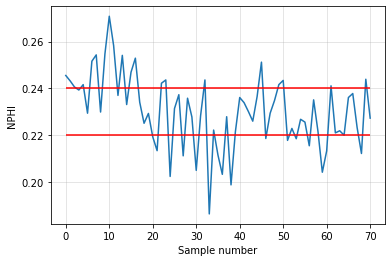porosity = 0.23

if porosity >= 0.24:
reservoir = 'Good'
elif 0.22 < porosity < 0.24:
reservoir = 'Medium'
else:
reservoir = 'Poor'

reservoir
'Medium'
for porosity in nphi[:5]:
if porosity >= 0.24:
reservoir = 'Good'
elif 0.22 < porosity < 0.24:
reservoir = 'Medium'
else:
reservoir = 'Poor'
print(reservoir)
Good
Good
Good
Medium
Good

good_reservoir_count = 0

for porosity in nphi[:10]:
if porosity >= 0.24:
good_reservoir_count += 1

good_reservoir_count
7
good_reservoir_count = 0

for porosity in nphi:
if porosity >= 0.24:
good_reservoir_count += 1

good_reservoir_count
20
# IGNORE this if it's too much of a stretch!
good_resevoir_lc = sum([1 for poro in nphi if poro > 0.24])
good_resevoir_lc
20

## An image speaks a thousand words¶

The python-visualization-landscape is vast and can be intimidating, no doubt you'll see many great examples during Transform 2022, but the place to start is usually matplotlib, you can reach the docs directly from with the jupyter notebook: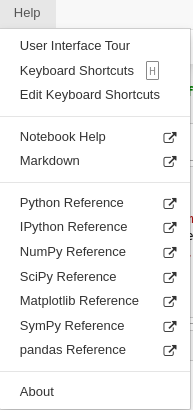import matplotlib.pyplot as plt
plt.plot(nphi)
[<matplotlib.lines.Line2D at 0x7f4e835d4d00>]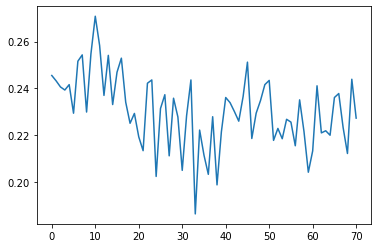plt.plot(nphi, 'o-', c='g')
[<matplotlib.lines.Line2D at 0x7f4e836a21f0>]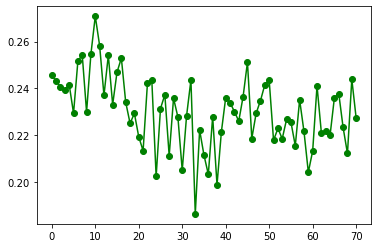fig, ax = plt.subplots()

ax.plot(nphi, 'o-', c='g', lw=0.4, markersize=2)
ax.set_ylabel('NPHI')
ax.set_xlabel('Sample number')
ax.set_title('Simple Porosity plot')
ax.grid(alpha=0.4)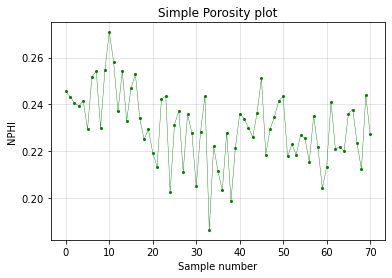Now we have created a plot, let's pull all of this together a single cell and save the figure:

import numpy as np
import matplotlib.pyplot as plt

nphi = data[:,1]

fig, ax = plt.subplots()

ax.plot(nphi, 'o-', c='g', lw=0.4, markersize=2)
ax.set_ylabel('NPHI')
ax.set_xlabel('Sample number')
ax.set_title('Simple Porosity plot')
ax.grid(alpha=0.4)

plt.savefig('./images/NPHI_plot.png')

plt.show()### Interactive plots¶

It is possible to add interactivity to plots in the Jupyter notebook using ipywidgets, without going through a full tutorial, here is a simple demo extending the plot we've been working with:

from ipywidgets import interact
import ipywidgets as widgets

@interact(high=widgets.FloatSlider(value=0.24, min=0.22, max=0.28, step=0.001, continuous_update=False),
low=widgets.FloatSlider(value=0.22, min=0.18, max=0.22, step=0.001, continuous_update=False)
)
def poro_high_low(high, low):
"""Create an interactive plot with ipywidgets."""
fig, ax = plt.subplots(figsize=(14, 6))
ax.plot(nphi, c='k', lw=1, markersize=6)
ax.plot(np.where(nphi > high, nphi, None), '^', c='r', lw=0.4, markersize=10)
ax.plot(np.where((nphi < high) & (nphi > low), nphi, None), 'o', c='g', lw=0.4, markersize=8)
ax.plot(np.where(nphi < low, nphi, None), 'v', c='b', lw=0.4, markersize=8)
ax.fill_between(np.arange(nphi.size), low, high, color='g', alpha=0.1)
ax.set_ylabel('NPHI')
ax.set_xlabel('Sample number')
ax.set_title('Simple Porosity plot')
ax.axhline(high, ls='--' ,c='r', label='high')
ax.axhline(low, ls='-.', c='b', label='low')
ax.legend()
ax.grid(alpha=0.4)

return None

## Wrap it up and do it again¶

Part of the beauty of code is its reusabilty, we've used functions already:

type(print)
builtin_function_or_method
sum([1, 2, 3])
6
# using plt.scatter


xs = [1, 2, 3, 4, 5, 6, 7, 8, 9, 10]
x2 = [x**2 for x in xs]
plt.scatter(xs, x2, c='r')
<matplotlib.collections.PathCollection at 0x7f4e831bf460>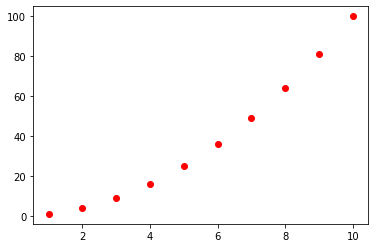# writing our own simple function


def my_adder(a, b):
return a + b
my_adder?
my_adder(45, 98)
143

### Writing actual equations in python¶

Remember this equation?

$\rho = \alpha {V_P}^{\beta}$

Let's turn it into a python function:

def gardner(vp, alpha=310, beta=0.25):
"""Evaluate gardner's relation.
Args:
vp (float): P-wave velocity
alpha (float-like): alpha scalar
beta (float-like): beta exponent
Returns:
rho (float): density
Source: https://subsurfwiki.org/wiki/Gardner%27s_equation
"""
return alpha * vp**beta
gardner(2766)
2248.147169062099

## Where to next?¶